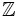# Multiplicative Euclidean norm

This article defines a property of a norm on a commutative unital ring obtained as the conjunction of two properties: multiplicative norm and Euclidean norm.
View a complete list of such conjunctions | View a complete list of properties of norms in commutative unital rings

## Definition

A multiplicative Euclidean norm is a function from a commutative unital ring to the nonnegative integers that satisfies the following two conditions:

## Examples

The absolute value on the ring of rational integers ($\mathbb{Z}$) is a multiplicative Euclidean norm. So is the square of the complex modulus, on the ring of Gaussian integers.

In general, the algebraic norm in a number field is multiplicative, and so is its restriction to the ring of integers.